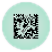# Matrix Sampler¶Samples a relation matrix.

## Signals¶

Inputs:

• Data

Data set.

Outputs:

• In-sample Data

Selected data.

• Out-of-the-sample Data

Remaining data.

## Description¶

This widget samples the input data and sends both the sampled and the remaining data to the output. It is useful for evaluating the performance of recommendation systems.1. Select the desired sampling method:
• rows (randomly samples entire matrix rows)
• columns (randomly samples entire matrix columns)
• rows and columns (samples from the entire matrix)
• entries (randomly samples individual matrix elements)
2. Select the proportion of the data you want at the output.
3. Press Apply to commit the changes.

## Example¶

Matrix Sampler widget samples data into two subsets: in-sample and out-of-the-sample data. This is useful if you want to check the accuracy of matrix reconstruction with Completion Scoring. Feed in-sample data into the Fusion Graph to reconstruct the matrix and then compare the results with out-of-the-sample data.